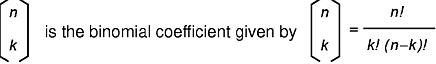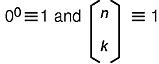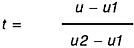[ Previous | Next | Contents | Glossary | Home | Search ]
OpenGL 1.1 for AIX: Reference Manual

## glMap1 Subroutine

### Purpose

Defines a 1-dimensional (1D) evaluator.

### Library

OpenGL C bindings library: libGL.a

### C Syntax

```void glMap1d(GLenum Target,
GLdouble u1,
GLdouble u2,
GLint Stride,
GLint Order,
const GLdouble *Points)```
```void glMap1f(GLenum Target,
GLfloat u1,
GLfloat u2,
GLint Stride,
GLint Order,
const GLfloat *Points)```

### Parameters

 Target Specifies the values that are generated by the evaluator. The following symbolic constants are accepted: GL_MAP1_VERTEX_3 GL_MAP1_VERTEX_4 GL_MAP1_INDEX GL_MAP1_COLOR_4 GL_MAP1_NORMAL GL_MAP1_TEXTURE_COORD_1 GL_MAP1_TEXTURE_COORD_2 GL_MAP1_TEXTURE_COORD_3 GL_MAP1_TEXTURE_COORD_4 u1, u2 Specify a linear mapping of u, as presented to glEvalCoord1, to u1, the variable that is evaluated by the equations specified by this subroutine. Stride Specifies the number of floats or doubles between the beginning of one control point and the beginning of the next one in the data structure referenced in Points. This allows control points to be embedded in arbitrary data structures. The only constraint is that the values for a particular control point must occupy contiguous memory locations. Order Specifies the number of control points. Must be positive. Points Specifies a pointer to the array of control points.

### Description

Evaluators provide a way to use polynomial or rational polynomial mapping to produce vertices, normals, texture coordinates, and colors. The values produced by an evaluator are sent to further stages of GL processing just as if they had been presented using the glVertex, glNormal, glTexCoord, and glColor subroutines, except that the generated values do not update the current normal, texture coordinates, or color.

All polynomial or rational polynomial splines of any degree (up to the maximum degree supported by the GL implementation) can be described using evaluators. These include almost all splines used in computer graphics, such as B-splines, Bezier curves, and Hermite splines.

Evaluators define curves based on Bernstein polynomials. Define p(t) as the following:

`Let p(t) = Bn0(t)R0 + Bn1(t)R1 + . . . + Bnn(t)Rn`

where Ri is a control point and Bni(t) is the ith Bernstein polynomial of degree:

`n (Order = n+1)`

See the figure:See the figure:The glMap1 subroutine is used to define the basis and to specify what kind of values are produced. Once defined, a map can be enabled and disabled by calling the glEnable and glDisable subroutines with the map name, one of the nine predefined values for the Target parameter. The glEvalCoord1 subroutine evaluates the 1D maps that are enabled. When glEvalCoord1 presents a value u, the Bernstein functions are evaluated using t, as in the following figure:The Target parameter specifies a symbolic constant that indicates what kind of control points are provided in the Points parameter, and what output is generated when the map is evaluated. It can assume one of the following nine predefined values:

 GL_MAP1_VERTEX_3 Each control point is three floating-point values representing x, y, and z. Internal glVertex3 subroutines are generated when the map is evaluated. GL_MAP1_VERTEX_4 Each control point is four floating-point values representing x, y, z, and w. Internal glVertex4 subroutines are generated when the map is evaluated. GL_MAP1_INDEX Each control point is a single floating-point value representing a color index. Internal glIndex subroutines are generated when the map is evaluated. However, the current index is not updated with the value of these glIndex subroutines. GL_MAP1_COLOR_4 Each control point is four floating-point values representing red, green, blue, and alpha (RGBA). Internal glColor4 subroutines are generated when the map is evaluated. However, the current color is not updated with the value of these glColor4 subroutines. GL_MAP1_NORMAL Each control point is three floating-point values representing the x, y, and z components of a normal vector. Internal glNormal subroutines are generated when the map is evaluated. However, the current normal is not updated with the value of these glNormal subroutines. GL_MAP1_TEXTURE_COORD_1 Each control point is a single floating-point value representing the s texture coordinate. Internal glTexCoord1 subroutines are generated when the map is evaluated. However, the current texture coordinates are not updated with the value of these glTexCoord subroutines. GL_MAP1_TEXTURE_COORD_2 Each control point is two floating-point values representing the s and t texture coordinates. Internal glTexCoord2 subroutines are generated when the map is evaluated. However, the current texture coordinates are not updated with the value of these glTexCoord subroutines. GL_MAP1_TEXTURE_COORD_3 Each control point is three floating-point values representing the s, t, and r texture coordinates. Internal glTexCoord3 subroutines are generated when the map is evaluated. However, the current texture coordinates are not updated with the value of these glTexCoord subroutines. GL_MAP1_TEXTURE_COORD_4 Each control point is four floating-point values representing the s, t, r and q texture coordinates. Internal glTexCoord4 subroutines are generated when the map is evaluated. However, the current texture coordinates are not updated with the value of these glTexCoord subroutines.

The Stride, Order, and Points parameters define the array addressing for accessing the control points. Points is the location of the first control point, which occupies one, two, three, or four contiguous memory locations, depending on which map is being defined. Order is the number of control points in the array. Stride tells how many float or double locations to advance the internal memory pointer to reach the next control point.

### Notes

As is the case with all GL subroutines that accept pointers to data, it is as if the contents of Points were copied by glMap1 before it returned. Changes to the contents of Points have no effect after glMap1 is called.

### Errors

 GL_INVALID_ENUM Target is not an accepted value. GL_INVALID_VALUE u1 is equal to u2. GL_INVALID_VALUE Stride is less than the number of values in a control point. GL_INVALID_VALUE Order is less than one or greater than GL_MAX_EVAL_ORDER. GL_INVALID_OPERATION The glMap1 subroutine is called between a call to glBegin and the corresponding call to glEnd.

### Associated Gets

Associated gets for the glMap1 subroutine are as follows. (See the glGet subroutine for more information.)

glGetMap

glGet with argument GL_MAX_EVAL_ORDER

glIsEnabled with argument GL_MAP1_VERTEX_3

glIsEnabled with argument GL_MAP1_VERTEX_4

glIsEnabled with argument GL_MAP1_INDEX

glIsEnabled with argument GL_MAP1_COLOR_4

glIsEnabled with argument GL_MAP1_NORMAL

glIsEnabled with argument GL_MAP1_TEXTURE_COORD_1

glIsEnabled with argument GL_MAP1_TEXTURE_COORD_2

glIsEnabled with argument GL_MAP1_TEXTURE_COORD_3

glIsEnabled with argument GL_MAP1_TEXTURE_COORD_4.

### Files

 /usr/include/GL/gl.h Contains C language constants, variable type definitions, and ANSI function prototypes for OpenGL.

### Related Information

The glBegin or glEnd subroutine, glColor subroutine, glEnable or glDisable subroutine, glEvalCoord subroutine, glEvalMesh subroutine, glEvalPoint subroutine, glGetMap subroutine, glIndex subroutine, glMap2 subroutine, glMapGrid subroutine, glNormal subroutine, glTexCoord subroutine, glVertex subroutine.

[ Previous | Next | Contents | Glossary | Home | Search ]